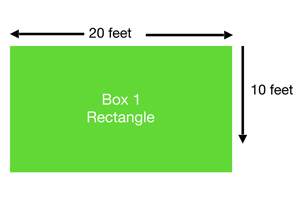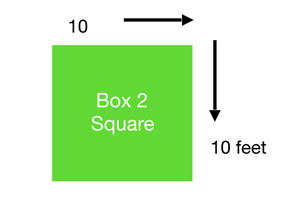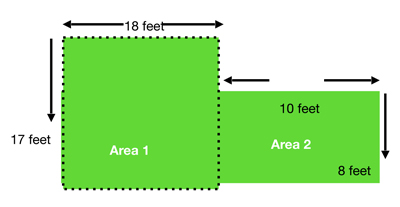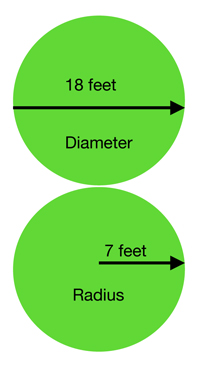### Areas of Squares and Rectangles

The simplest (and most commonly used) area calculations are for squares and rectangles.

To find the area of a rectangle multiply its height by its width.

For a square you only need to find the length of one of the sides (as each side is the same length) and then multiply this by itself to find the area. This is the same as saying length2 or length squared.

It is good practice to check that a shape is actually a square by measuring both sides.
For example your lawn may look same length on both side or square however when you measure it each side is different. Alway use the largest of the 2 numbers so you don’t short change yourself on materials.A typical backyard is often more complex than a simple rectangle or square. The following diagrams will help you breakdown your measurements by section.To determine the square feet of this shape you can divide the area into two squares, then simply add the two areas together.

Area 1 = 18ft x 17ft= Total 306 sq.ft.

Area 2 = 10ft x 8ft =Total 80 sq.ft.

Total area of Area 1 + 2: 306+80 = 386 sq.ft.

### How to calculate areas of circlesThe diameter of a circle is the length of a straight line from one side of the circle to the other that passes through the central point of the circle. The diameter is twice the length of the radius (diameter = radius × 2)

The radius of a circle is the length of a straight line from the central point of the circle to its edge. The radius is half of the diameter. (radius = diameter ÷ 2)

You can measure the diameter or radius at any point around the circle – the important thing is to measure using a straight line that passes through (diameter) or ends at (radius) the centre of the circle.

When measuring circles it’s easier to measure the diameter, then divide by 2 to find the radius.

The radius is needed to work our the area of a circle.The formula is:

circle area = πR2.

This means:

π = Pi is a constant that equals 3.142.

R = is the radius of the circle.

Therefore a circle with a radius of 7 ft, has an area of:

3.142 × 9 × 9 = 153.958 square feet or 154 sq.ft.

circle with a diameter of 18 ft has an area:

First we work out the radius (18ft ÷ 2 = 9 ft )

Then apply the formula:

3.142 × 9 x 9 = 254.502 square feet

The area of a circle with a diameter of 18 feet is 254.5 sq.ft.

CAUTION: Your circle square feet is not what you need to purchase artificial turf. Ask us for
help to determine your actual requirements.

## Landscaping Fill Calculator

 length footinchyardmetercentimeter width footinchyardmetercentimeter thickness or height footinchyardmetercentimeter Quantity

### Request a Quote

• Date Format: MM slash DD slash YYYY
• This field is for validation purposes and should be left unchanged.